Standard Deviation - Measures of Dispersion, Business Mathematics & Statistics

# Standard Deviation - Measures of Dispersion, Business Mathematics & Statistics - Business Mathematics and Statistics - B Com

Standard Deviation :

In calculating mean deviation we ignored the algebraic signs, which is mathematically illogical. This drawback is removed in calculating standard deviation, usually denoted by ‘ σ ’ (read as sigma)

Definition : Standard deviation is the square root of the arithmetic average of the squares of all the deviations from the mean. In short, it may be defined as root-mean-square deviation from the mean.

If x is the mean of x1 , x2 , ……., xn , then σ is defined by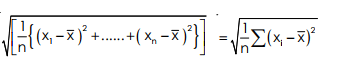Different formulae for computing s. d. (a) For simple observations or variates.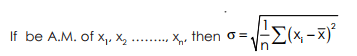(b) For simple or group frequency distribution For the variates x1 , x2 , x3 , ……., xn , if corresponding frequencies are f1 , f2 , f3 , ….., fn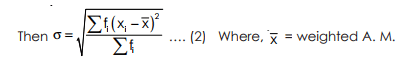Note : If variates are all equal (say K), then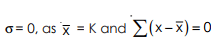Example 49: For observations 4, 4, 4, 4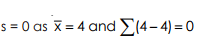Short cut method for calculating s.d. If x (A. M.) is not an integer, in case (1), (2) ; then the calculation is lengthy and time consuming. In such case, we shall follow the following formulae for finding s.d.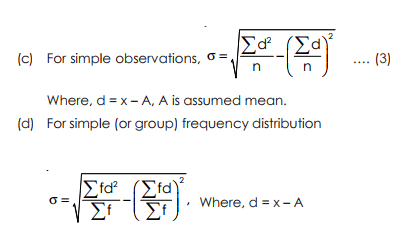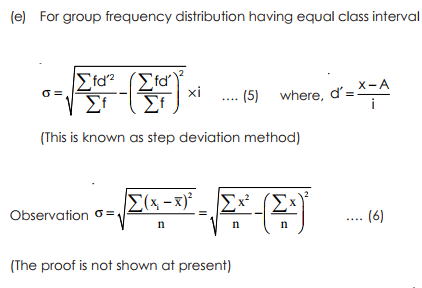Note : Formula (3) may be written as, for step deviation where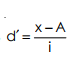Computation for Standard Deviation :
(A) For individual observations computation may be done in two ways :
(a) by taking deviations from actual mean. Steps to follow––

(1) Find the actual mean, i.e.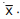.
(2) Find the deviations from the mean, i.e., d.
(3) Make squares of the deviations, and add up, i.e.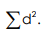.
(4) Divide the addition by total number of items, i.e., find d2 / n and hence make square root of it.

(b) by taking deviations from assumed mean. Steps to follow––

by taking deviations from assumed mean. Steps to follow––
(1) Find the deviations of the items from an assumed mean and denote it by d find also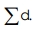.
(2) Square the deviations, find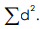.
(3) Apply the following formula to find standard deviation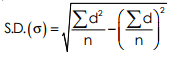Example 50 : Find s.d. of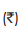7, 9, 16, 24, 26. Calculation of s.d. by methods (a) Taking deviations of Sum (b) Taking deviations from Assumed Mean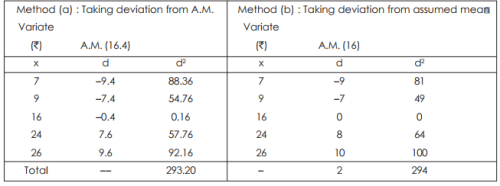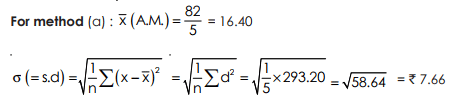Here the average or A.M. 16.40 and the variates deviate on an average from the A.M. by ` 7.66. For method (b) : Let A (assumed mean) = 16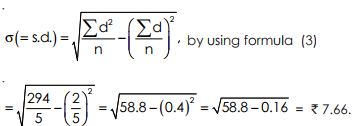Note : If the actual mean is in fraction, then it is better to take deviations from an assumed mean, for avoiding too much calculations. (B) For discrete series (or Simple Frequency Distribution). There are three methods, given below for computing Standard Deviation. (a) Actual Mean, (b) Assumed Mean, (c) Step Deviation. For (a) the following formula are used. This method is used rarely because if the actual mean is in fractions, calculations take much time.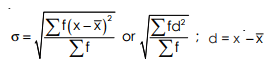(In general, application of this formula is less) For (b), the following steps are to be used :–
(i) Find the deviations (from assumed mean), denote it by d.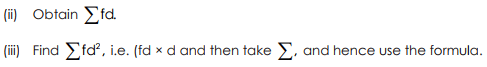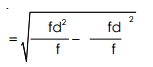Example 51 : Find the Standard deviation of the following series :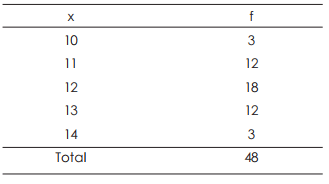Solution :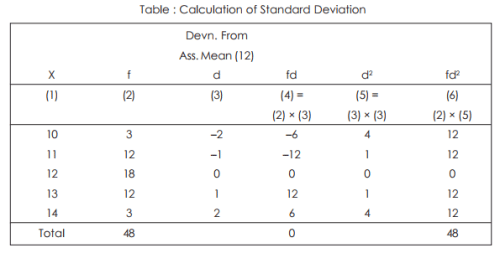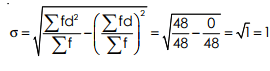For (c) the following formula is used.
The idea will be clear from the example shown below :

Formula is,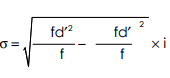where d′ = step deviation, i = common factor.

Example 52: Find the standard deviation for the following distribution :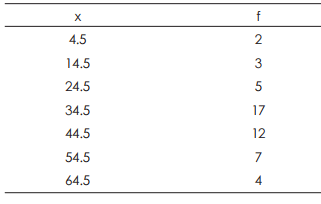Solution :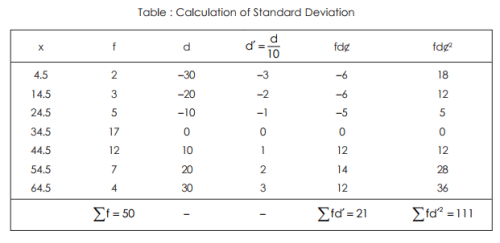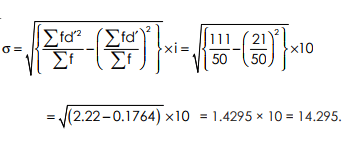(C) For Continuous Series (or group distribution) :
Any method discussed above (for discrete series) can be used in this case. Of course, step deviation method is convenient to use. From the following example, procedure of calculation will be clear

. Example 53 : Find the standard deviation from the following frequency distribution.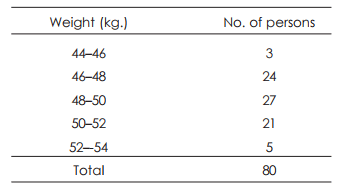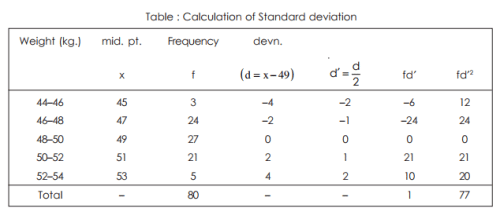Let A (assumed mean) = 49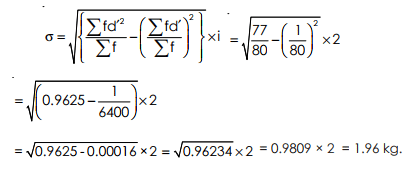MATHEMATICAL PROPERTIES OF STANDARD DEVIATION :
Combined Standard Deviation.

We can also calculate the combined standard deviation for two or more groups, similar to mean of composite group. The required formula is as follows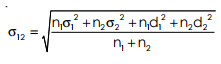where σ12 = combined standard deviation of two groups.
σ1 = standard deviation of 1st group.
σ2 = standard deviation of 2nd group.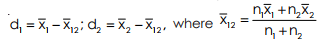For Three Groups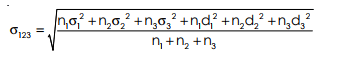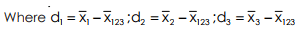Example 54 : Two samples of sizes 40 and 50 respectively have the same mean 53, but different standard deviations 19 and 8 respectively. Find the Standard Deviations of the combined sample of size 90.

Solution: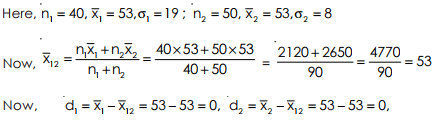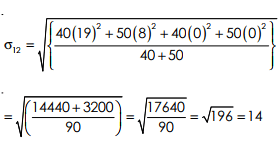The document Standard Deviation - Measures of Dispersion, Business Mathematics & Statistics | Business Mathematics and Statistics - B Com is a part of the B Com Course Business Mathematics and Statistics.
All you need of B Com at this link: B Com

115 videos|142 docs

## FAQs on Standard Deviation - Measures of Dispersion, Business Mathematics & Statistics - Business Mathematics and Statistics - B Com

 1. What is standard deviation and why is it important in business mathematics and statistics?Ans. Standard deviation is a measure of dispersion that quantifies the amount of variability or spread in a data set. It provides information about how spread out the data points are from the mean. In business mathematics and statistics, standard deviation is important because it helps in analyzing and understanding the risk and volatility associated with various business activities, such as investment returns, sales data, or production output.
 2. How is standard deviation calculated and what is the formula?Ans. Standard deviation is calculated by taking the square root of the variance. The formula for calculating standard deviation is as follows: Standard Deviation = √(Σ(xi - x̄)² / N) Where: - Σ represents the sum of - xi represents each individual data point - x̄ represents the mean of the data set - N represents the total number of data points
 3. What does a high standard deviation indicate in business mathematics and statistics?Ans. A high standard deviation indicates that the data points in a data set are spread out over a wider range and have greater variability. In business mathematics and statistics, a high standard deviation suggests that there is a higher level of risk or uncertainty associated with the data. For example, if the standard deviation of investment returns is high, it implies that the returns are more volatile and unpredictable, which may be perceived as riskier by investors.
 4. How does standard deviation help in comparing data sets?Ans. Standard deviation helps in comparing data sets by providing a measure of dispersion or spread. By comparing the standard deviations of two or more data sets, we can determine which data set has more variability. If one data set has a higher standard deviation compared to another, it indicates that the data points in that set are more spread out. This comparison can be useful in various business scenarios, such as comparing the sales performance of different products or comparing the production output of different factories.
 5. Can standard deviation be negative and what does it mean?Ans. No, standard deviation cannot be negative. Standard deviation is always a non-negative value because it represents a measure of spread or variability. It tells us how far the data points are from the mean, but it does not have a direction. A negative value would imply that the data points are on average below the mean, which contradicts the concept of standard deviation. Therefore, standard deviation is always reported as a positive value or zero if all the data points are the same.

115 videos|142 docsExplore Courses for B Com examSignup to see your scores go up within 7 days! Learn & Practice with 1000+ FREE Notes, Videos & Tests.
10M+ students study on EduRev
Track your progress, build streaks, highlight & save important lessons and more!
Related Searches

,

,

,

,

,

,

,

,

,

,

,

,

,

,

,

,

,

,

,

,

,

,

,

,

;